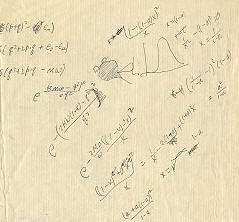## Friday, 18 July 2008

### EASY????Some calculations are easier than you think! I’m drawn to math that can be done on a napkin at a restaurant. Quick mental calculations and methods of approximation that give you the ball park quick answer…”It’s about …blah.” and then I try to look as if I don’t think I’m really clever. I had a couple of these come up recently, so I thought I would share…

One was at a backyard barbecue…an electrical engineer, a graduate physics student, and a math teacher, beers in hand as the hamburgers burned and the hot dogs turned to charcoal… One had just come back from New York City and pointed out that the Empire State bldg. was 1224 feet high to the upper observatory… then someone brought up the old conjecture that a penny dropped from the Empire State building would kill you. The engineer suggests that in truth the penny would reach some terminal velocity that would keep it from actually killing anyone. The physics student suggests that it would not be too hard to figure out using differential equations if we just ignored the air resistance, but he didn’t have his calculator (which apparently is his main resource for doing integration). The math teacher offers, “Well, with only high school math and physics, and a little mental calculation, it should be about 280 feet per second.”

A long silence, followed by “How did you get that number?” So I explained. In physics the big ideas often provide great simplifications…and in this case the big idea was the conservation of energy laws…. The total energy remains constant. The Penny on the 102nd floor has a potential energy equal to its weight times its height. When it hits the ground the potential energy has all been converted to kinetic energy (and a very small amount of heat due to air friction which I ignore) . Epot= m g h and Ekin = ½ m v2. Cancel the mass on each side and we have gh = ½ v2. If we use the common value of 32 ft/sec2 for the gravitational force, then we can simplify this all down to v2= 64 h.. or taking square roots, we get v= 8 times the square root of h…. Now the square root of 1224 is about the same as the square root of 1225 which is 35 (one of those multiplication tricks I teach my students each year) and 8 x 35 = 280, so the answer is about 280 ft/second.

They both look incredulous… so I offer a second approach… OK, an object dropped from rest will fall 16t2 feet in t seconds. To fall 1224 seconds it would take t=sqrt(1224/16) and taking the square root of top and bottom we get 35/4 seconds… OK, so velocity is 32 times t, so 32 times 35/4 = (wait for it…. ) 8 x 35 = 280 again… “Easier than you thought… right

The second event corrected a misconception I have had for years. I have mentioned earlier that one of my preoccupations on the road is looking at mile markers and license plate numbers and trying to factor them. I know all the easy tricks for divisibility by small numbers, except seven…. The rules for divisibility by seven always seemed harder than just dividing by seven, so I was surprised to read a note from a guy who seems to have invented (I never saw it before) an easy way to test divisibility by seven

Without going into the derivation, here is the rule. Take the number, call it N, and think of it as 10A + B. For example if the number is 2345 the A = 234 and the B= 5. Now the method is just to calculate A-2B and if that answer is divisible by seven, so is the original. And if you don’t know, just reapply the same rule again. For example 2345 is divisible by 7 only if 234-10 = 224 is also….which can be applied again with 22-8 = 14… ohh….. I KNOW that is a multiple of seven, so 2345 is also a multiple of seven, in fact it is 7 x 335.

This is getting a little long, so on a later day I’ll explain how he found it, and even show you a method I found for testing 13 which is almost as easy, and another for testing divisibility by 17… as the tv folks say….stay tuned